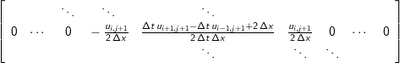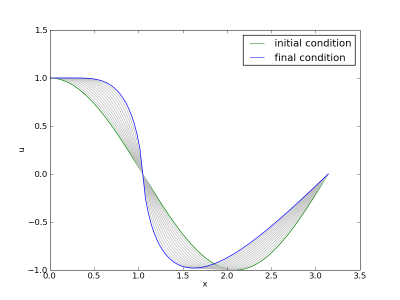## Friday, June 19, 2009

### Central Schemes and Discontinuities

I've been posting lately on solving Burgers' equation on moving grids, but haven't said much about difficulties involved in properly approximating the non-linear term involving the spatial derivative. A naive approach to solving Burgers' equation with central differences leads to problems, termed "blow-up" by early practitioners doing climate modeling. Boyd discusses this problem as it relates to spectral methods.

A backward-time centered-space (BTCS) discretization of Burgers equation leads to a non-linear equation for each grid point that depends on it's nearest neighbors in the spatial coordinate and it's predecessor in the time coordinate. Backward time:Centered space:Resulting discrete Burgers' equation:We can use a Newton method to solve the resulting system, and to do that we'll need the Jacobian:To solve for the update in each iteration of the Newton's method, we'll generally need an expression for the action of the Jacobian on the update vector:All of these symbolic manipulations of the governing equation can be done easily in Maxima (see here and here and here for examples).

Here's a graph of several time integration steps of the BTCS Burgers equation starting with part of a cosine:This shows the characteristic behaviour of non-linear wave equations known as wave steepening, which causes the formation of shock waves (discontinuities). Unfortunately, if we take just a few more time-steps we get the beginnings of "blow-up":Another interesting case is a small advecting step shown below.In this case the size of the discontinuity is much smaller compared to the grid resolution, so the solution does not blow-up, but some weird things happen. Burgers' equation has one eigenvalue, or wave speed. All of the waves should travel in the plus x-direction, because u is positive everywhere in this case. That's not what we see though. We see the main step travel in the proper direction (after some smearing due to the first order time integration), but we see wiggly errors going in the opposite direction. Because the central differencing scheme does not respect the eigenvalues of the problem (the way an upwind scheme would), we introduced a new dispersive error wave that travels the wrong way.